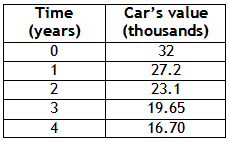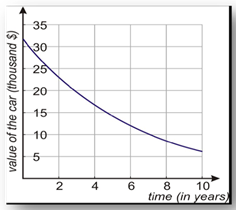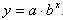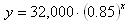Application
Example 2

The cost of a new car is \$32,000. It depreciates at a rate of 15% per year. This means that it loses 15% of each value each year.

a) Draw the graph of the car's value versus the time in years.
b) Find the formula that gives the value of the car in terms of time.
c) Find the value of the car when it is four years old.

Step 1. Make a table of values to represent the function.Step 2. Plot the points to make a graph.Step 3. Write a model for the exponential decay.

Remember that the general formula for an exponential function isa is the initial value, so a = 32,000.

Since the car is decreasing from 100% at a rate of 15%, b = 100%-15% = 85% = 0.85.

Therefore, the equation isStep 4. Determine the value of the car after four years.

Since x represents the number of years, let x = 4.

y = 32,000 · (0.85)4 = \$16,704.
.